# Metal Catalog

Metal catalog, conductors

Use Antenna Toolbox™ functions to select and customize different metals for designing an antenna. Explore different conductor materials and model the conductivity and thickness of the metal to optimize your antenna design and reduce resistive losses.

Metal Catalog

 PECσ = Infd = 0 Copper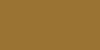σ = 59.6e06 S/md = 3.556e-05 m Aluminium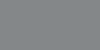σ = 37.7e06 S/md = 7.62e-04 m Gold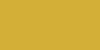σ = 41.1e06 S/md = 2e-07 m Silver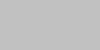σ = 63e06 S/md = 2e-07 m Custom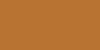σ = User definedd = User defined Zinc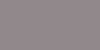σ = 16.9e06 S/md = 1.016e-04 m Tungstenσ = 17.9e06 S/md = 2e-07 m Lead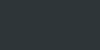σ = 4.55e06 S/md = 2e-07 m Iron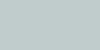σ = 10e06 S/md = 2e-07 m Steel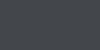σ = 6.99e06 S/md = 0.68e-03 m Brassσ = 15.9e06 S/md = 0.68e-03 m

## Apps

 Antenna Designer Design, visualize, and analyze antennas Antenna Array Designer Design, visualize, and analyze arrays (Since R2019b)

## Functions

 `metal` Conductor material (Since R2021a) `MetalCatalog` Catalog of metals (Since R2021a)